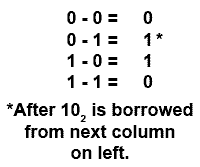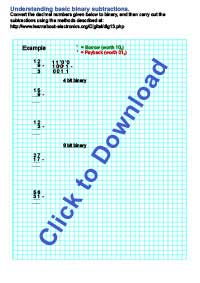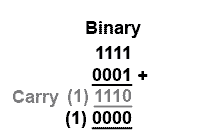# Binary Arithmetic

• After studying this section, you should be able to:
• Understand the rules used in binary calculations.
• • Subtraction.
• • Use of carry, borrow & pay back.
• Understand limitations in binary arithmetic.
• • Word length.
• • Overflow.

Arithmetic rules for binary numbers are quite straightforward, and similar to those used in decimal arithmetic. The rules for addition of binary numbers are:##### Fig. 1.3.1 Rules for Binary Addition

Notice that in Fig. 1.3.1, 1+1 = (1)0 requires a ‘carry’ of 1 to the next column. Remember that binary 102 = 210 decimal

### Example:##### Fig. 1.3.2 Simple Binary Addition

Binary addition is carried out just like decimal, by adding up the columns, starting at the right and working column by column towards the left.##### Fig. 1.3.3 Binary Addition with Carry

Just as in decimal addition, it is sometimes necessary to use a ‘carry’, and the carry is added to the next column. For example, in Fig. 1.3.3 when two ones in the right-most column are added, the result is 210 or 102, the least significant bit of the answer is therefore 0 and the 1 becomes the carry bit to be added to the 1 in the next column.

## Binary Subtraction

The rules for subtraction of binary numbers are again similar to decimal. When a large digit is to be subtracted from a smaller one, a ‘borrow’ is taken from the next column to the left. In decimal subtractions the digit ‘borrowed in’ is worth ten, but in binary subtractions the ‘borrowed in’ digit must be worth 210 or binary 102.

After borrowing from the next column to the left, a ‘pay back’ must occur. The subtraction rules for binary are quite simple even if the borrow and pay back system create some difficulty. Depending where and when you learned subtraction at school, you may have learned a different subtraction method, other than ‘borrow and payback’, this is caused by changing fashions in education. However any method of basic subtraction will work with binary subtraction but if you do not want to use ‘borrow and payback’ you will need to apply your own subtraction method to the problem.## Binary Subtraction Rules

The rules for binary subtraction are quite straightforward except that when 1 is subtracted from 0, a borrow must be created from the next most significant column. This borrow is then worth 210 or 102 because a 1 bit in the next column to the left is always worth twice the value of the column on its right.

Fig. 1.3.5 shows how binary subtraction works by subtracting 510 from 1110 in both decimal and binary. Notice that in the third column from the right (22) a borrow from the (23) column is made and then paid back in the MSB (23) column.

Note: In Fig 1.3.5 a borrow is shown as 10,and a pay back is shown as 01. Borrowing 1 from the next highest value column to the left converts the 0 in the 22 column into 102 and paying back 1 from the 22 column to the 23 adds 1 to that column converting the 0 to 012.##### Fig. 1.3.5 Binary Subtraction

Once these basic ideas are understood, binary subtraction is not difficult, but does require some care. As the main concern in this module is with electronic methods of performing arithmetic however, it will not be necessary to carry out manual subtraction of binary numbers using this method very often. This is because electronic methods of subtraction do not use borrow and pay back, as it leads to over complex circuits and slower operation. Computers therefore, use methods that do not involve borrow. These methods will be fully explained in Number Systems Modules 1.5 to 1.7.

## Subtraction ExerciseJust to make sure you understand basic binary subtractions try the examples below on paper. Don’t use your calculator, click the image to download and print the exercise sheet. Be sure to show your working, including borrows and paybacks where appropriate. Using the squared paper helps prevent errors by keeping your binary columns in line. This way you will learn about the number systems, not just the numbers.## Limitations of Binary Arithmetic

Now back to ADDITION to illustrate a problem with binary arithmetic. In Fig. 1.3.6 notice how the carry goes right up to the most significant bit.

This is not a problem with this example as the answer 10102 (1010) still fits within 4 bits, but what would happen if the total was greater than 1510?##### Fig. 1.3.7 The Overflow Problem

As shown in Fig 1.3.7 there are cases where a carry bit is created that will not fit into the 4-bit binary word. When arithmetic is carried out by electronic circuits, storage locations called registers are used that can hold only a definite number of bits. If the register can only hold four bits, then this example would raise a problem. The final carry bit is lost because it cannot be accommodated in the 4-bit register, therefore the answer will be wrong.

To handle larger numbers more bits must be used, but no matter how many bits are used, sooner or later there must be a limit. How numbers are held in a computer system depends largely on the size of the registers available and the method of storing data in them, however any electronic system will have a way of overcoming this ‘overflow’ problem, but will also have some limit to the accuracy of its arithmetic.

Top of Page